# SSAT Middle Level Math : How to find perimeter of a triangle

## Example Questions

2 Next →

### Example Question #1 : How To Find The Perimeter Of A Triangle

Find the perimeter of a triangle with sides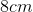,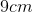, and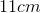.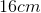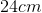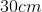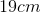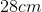Explanation:

Perimeter involves adding up all of the sides of the shapes; in this case, it's a triangle. Perimeter equals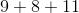. Therefore, the answer is.

### Example Question #2 : How To Find The Perimeter Of A Triangle

A right triangle has legsfeet andfeet long. What is its perimeter, in inches?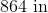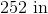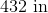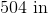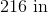Explanation:

The length of the hypotenuse of the triangle can be found using the Pythagorean Theorem. Substitute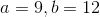: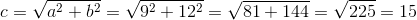feet

Its perimeter is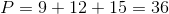feet. Multiply by 12 to get the perimeter in inches: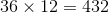inches

### Example Question #3 : How To Find The Perimeter Of A Triangle

An equilateral triangle has perimeter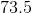centimeters. How long is one side?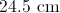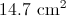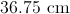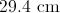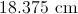Explanation:

An equilateral triangle has three sides of equal measure, so divide its perimeter by: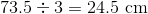### Example Question #11 : How To Find Perimeter Of A Triangle

Find the perimeter of an equilateral triangle with a base of 11in.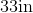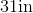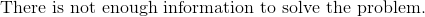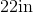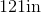Explanation:

To find the perimeter of a triangle, we will use the following formula: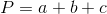where a, b, and c are the lengths of the sides of the triangle.

Now, we know the base of the equilateral triangle is 11in.  Because it is an equilateral triangle, all sides are equal.  Therefore, all sides are 11in.

So, we can substitute.  We get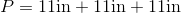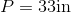### Example Question #12 : How To Find Perimeter Of A Triangle

Which of these choices can the perimeter of an isosceles triangle possibly be if you know that two sides have lengths 12 and 13?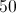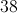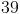Explanation:

An isosceles triangle has two sides of equal length. If two sides of an isoscelese triangle are of length 12 and 13, then the third must be of length 12 or 13.

This makes the perimeter either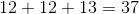or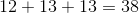Of our choices, we choose 38.

2 Next →